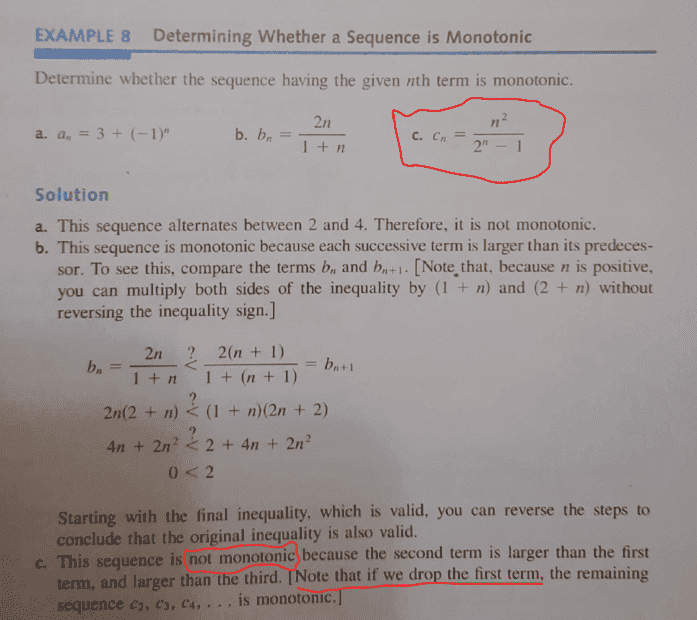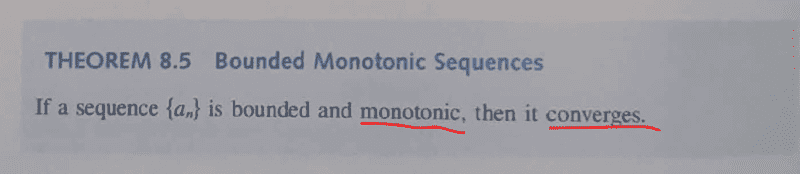# Bounded and monotonic sequences - Convergence

• chwala
In summary, the conversation discusses the relevance of determining whether a sequence is monotonic and bounded in order to prove convergence. It is noted that a sequence does not have to be monotonic to converge, and that finding the limit of a sequence directly may be difficult or impossible. The conversation also provides an example of using a monotonic approach to prove convergence and determine the limit of a sequence.

#### chwala

Gold Member
Homework Statement
see attached
Relevant Equations
Bounded and monotonic sequences - Convergence
I would like some clarity on the highlighted part. My question is, consider the the attached example ##(c)##, This sequence converges ( by using L'Hopital's rule)...now my question is, the sequence is indicated on text as not being monotonic...very clear. Does it imply that if a sequence is not monotonic, then it would not converge? In any case, what is the importance or rather the relevance of determining whether a sequence is monotonic (finding upper bounds and lower bounds) or not? Why not just take limit for the sequence and have the job done?chwala said:
Homework Statement:: see attached
Relevant Equations:: Bounded and monotonic sequences - Convergence

I would like some clarity on the highlighted part. My question is, consider the the attached example ##(c)##, This sequence converges ( by using L'Hopital's rule)...now my question is, the sequence is indicated on text as not being monotonic...very clear. Does it imply that if a sequence is not monotonic, then it would not converge?
No. A sequence does not have to be monotonic in order to converge.
chwala said:
In any case, what is the importance or rather the relevance of determining whether a sequence is monotonic (finding upper bounds and lower bounds) or not? Why not just take limit for the sequence and have the job done?
It might be extremely difficult and even impossible to find the limit of a sequence directly. A theorem that tells you a sequence must converge without having to find that limit explicity is an extremely useful mathematical idea.

PeroK said:
No. A sequence does not have to be monotonic in order to converge.

It might be extremely difficult and even impossible to find the limit of a sequence directly. A theorem that tells you a sequence must converge without having to find that limit explicity is an extremely useful mathematical idea.
Cheers Perok! Noted...

PeroK said:
No. A sequence does not have to be monotonic in order to converge.

It might be extremely difficult and even impossible to find the limit of a sequence directly. A theorem that tells you a sequence must converge without having to find that limit explicity is an extremely useful mathematical idea.
I would appreciate an example of a sequence ...that would have it easier using a monotonic approach in determining convergence due to the fact that it wouldn't be any easier to use limits...

Just to emphasize: The statement is that if it is bounded and monotonic, then it converges. It is not an if and only if, ie, the implication only goes one way.

•chwala and PeroK
chwala said:
I would appreciate an example of a sequence ...that would have it easier using a monotonic approach in determining convergence due to the fact that it wouldn't be any easier to use limits...
It's often useful when dealing with specific recursive sequences.

Also, you may have a sequence generated as part of a proof that you can show is bounded and monotonic. You may not have a specific sequence and so there would be no way to calculate an actual limit. The important thing is that you know that a limit exists.

I'll look for an example this afternoon.

•chwala
chwala said:
I would appreciate an example of a sequence ...that would have it easier using a monotonic approach in determining convergence due to the fact that it wouldn't be any easier to use limits...
Define ##x_n:=\dfrac{a_{2n-1}}{a_{2n}}## with the sequence ##a_n = 6a_{n-2} - a_{n-4}## for ##n > 4,## with ##a_1=1, a_2=0, a_3=3, a_4=2.## Prove monotony, boundedness, and determine the limit of ##(x_n)_{n\in \mathbb{N}}.##

I know the results, but not what is easiest to do.

fresh_42 said:
Define ##x_n:=\dfrac{a_{2n-1}}{a_{2n}}## with the sequence ##a_n = 6a_{n-2} - a_{n-4}## for ##n > 4,## with ##a_1=1, a_2=0, a_3=3, a_4=2.## Prove monotony, boundedness, and determine the limit of ##(x_n)_{n\in \mathbb{N}}.##

I know the results, but not what is easiest to do.
Wawawa...I will need to check on this fresh_42...

first attempt;

I want to get the sequence right, given ##(x_n)_{n\in \mathbb{N}}## and ##n > 4,## then we shall have ##x_5:=\dfrac{a_{10-1}}{a_{10}}##...correct?⇒##x_6:=\dfrac{a_{12-1}}{a_{12}}##...am reading something on cauchy criterion,... i just need to understand what i am supposed to do and my brain can work on it...still reading oops...second attempt;

Given,
##a_n = 6a_{n-2} - a_{n-4}## for ##n > 4,## with ##a_1=1, a_2=0, a_3=3, a_4=2.##
then it follows that,
##a_5= 6a_3 - a_1=18-1=17 ##
##a_6 = 6a_4 - a_2 =12-0=12##
##a_7 = 6a_5 - a_1 =102-3=99##
##a_8 = 6a_6 - a_4=72-2=70##
##a_9 = 6a_7 - a_5=594-17=577##
##a_{10}= 6a_8 - a_6=420-12=408##
##a_{11}= 6a_9 - a_7=3462-99=3363##
##a_{12}= 6a_{10} - a_8=2448-70=2378##
therefore, our ##x_n## will be given by;
##x_5:=\dfrac{a_{10-1}}{a_{10}}=\dfrac{577}{408}=1.414215686...## then it follows that our ##x_{n+1}## will be given by;
##x_6:=\dfrac{a_{12-1}}{a_{12}}=\dfrac{3363}{2378}=1.414213625...##
...

Am i doing the correct thing here? or am i supposed to use induction hypothesis to prove boundedness and monotonicity ( Monotone convergence Theorem)?then conclude using limits... Cheers...

Last edited: